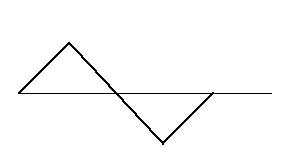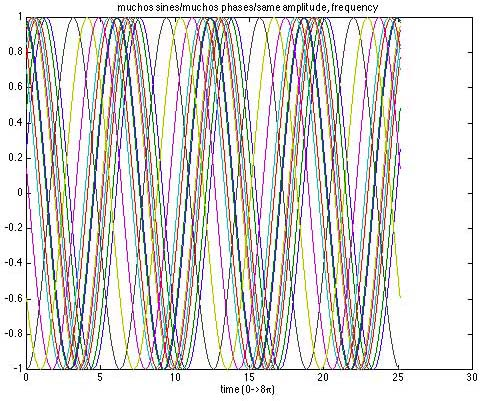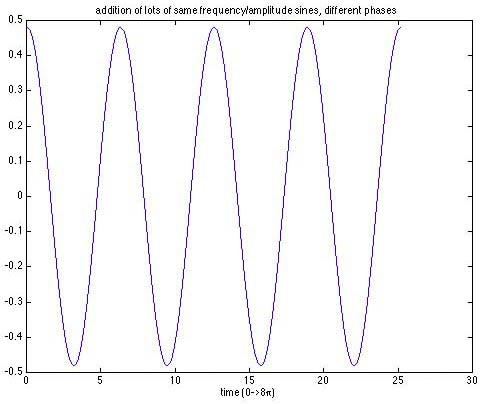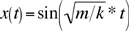# The Mathematics of Pure Tones

### Tuning Forks and the Basics of Sound

We’ve now seen a few audiograms and sound sure can look messy. As scientists we want to look for the simplest kinds of sounds and then build any sound out of our fundamental building blocks.

Just as chemistry tries to understand the properties of a substance by looking at the molecular structure, which in turn is studied by considering the individual atoms, we want to understand sound by finding "atoms of sound," understanding these, and then combining the atoms into molecules of sound, and finally the molecules combine to form general sounds, like a symphony, a voice, or the wind rustling through the leaves.

What should these pure sounds be? Let’s think a bit about music. Most of us have seen a musical score. At its most basic level, the score lays out a sequence of notes to be played and the duration that the notes need to be held. So, running left to right is time, and up and down is pitch (middle C, A sharp, etc.). The "pure" tones are the notes. Notes are realized differently on each instrument, but in fact, they are characterized as particular kinds of sound waves that occur at specific frequencies. We’ll explain this idea now.

Different instruments realize these notes in different ways—an A on a guitar sounds different from an A on a trombone or a flute. That’s because the physics of a plucked string is different from the physics of a open-ended tube. As a way of understanding pure tones, or our atoms of sound, the simplest model is the tuning fork.

We ring a tuning fork by whacking it against something and then standing it up and allowing the vibrations of the tines to disturb the surrounding air, sending the resulting sound to the waiting ears of everyone in the vicinity.

The tines move back and forth, sending the air molecules to your ear, where they go crashing against your eardrum. So, what might the audiogram look like?

Well, let’s concentrate our attention on the tip of one of the tines. In fact, imagine that we put a drop of glow-in-the-dark paint on the tip, turn the lights off, and follow the glowing dot. We see it move back and forth regularly (does a stiffer fork vibrate faster or slower than a "flabby" fork?). The air molecules then get sent away, and our ear drums more or less follow the same pattern. So, one possibility is something like this:Figure 1

Notice the regular up and down. It is a periodic wave, in the sense that there is a simple pattern that is repeated over and over again. The number of full copies of the pattern that fit in a single second is the frequency of the periodic function, and as we’ve already remarked, is measured in Hertz, or cycles per second. The duration of a single copy of the basic pattern is called the period of the periodic function. The period and frequency are related by

period = 1/frequency

Now one possible drawback of the "triangle" wave (we call it that because the wave forms a triangle above and below the x-axis) is that it isn’t smooth. The graph makes it look like as the tine reaches its maximal displacement, that it immediately whips back in the other direction. Our experience is that the change of direction is a bit more subtle than that—that there is a gradual slowing down as the apex is reached, and then just for a moment, it’s as if the tine stops, and then a gradual speeding up, and so on and so on... So, maybe instead, the graph is more like a smoothed out version of the triangle, maybe something like this:Figure 2

Some of you may recognize this as the graph of the sine function! In fact, this is the right graph, and before we convince you of that mathematically, it’s worth pointing out a few things about this graph.

We’ve already talked about a sine’s frequency, or equivalently, its period. Figure 3 shows a few different kinds of sine functions of differing frequencies (but the same amplitude) all superimposed over one another. Higher frequency means the peaks and troughs are squeezed more closely together.

The following picture shows a lot of sine waves, all at different frequencies, but at only one amplitude.Figure 3

Perceptually, higher frequency (usually) means a higher pitch, although in fact this is sort of complicated and we’ll discuss that later in Section 1.4.

A few other things about these graphs: There are other things that could have been varied (besides the frequency) in making the pictures. We could have also varied the height (or equivalently, the depth) of the wave. This parameter is called the amplitude of the wave. Also, there is an arbitrary nature to where in time we started to measure the displacement. If we had started a moment later or earlier, we would have obtained a shifted version of this picture, though we’d still have a sine wave.

Figure 5 shows what happens when we have a lot of sine waves, all at the same frequency and all at the same amplitude, but at different phases.Figure 4

Strangely enough, when we add up all those amplitude/frequency-similar-but-phase-different sine waves, we get—you guessed it—one pretty ordinary sine wave!Figure 5

This phase parameter is called a phase shift. To conclude, any sinusoidal wave is determined by three parameters: frequency, amplitude, and phase shift. We’ll be studying how the values of these parameters affect what we hear.

## Getting to the Math

Newton's second law states that the force felt by an object in motion is in proportion to the acceleration experienced by the object. For example, think about driving in your car—you and the contents of your fine automobile are the objects in motion. You are speeding down the interstate, brimming coffee cup in the cup holder and compact discs on the seat next to you. You’re cruising at 80 mph, so no acceleration, and life is good. You’re slouched in your seat, coffee isn’t spilling, CDs stay in place. Suddenly, you pass a state trooper hidden in the median. You hit the brakes, causing a deceleration (a negative acceleration), and now the calm of your car is disturbed. CDs go sliding to the floor, coffee sloshes against the side of the cup, and you find yourself thrown forward a bit in your seat. Everything is experiencing a force! The harder you brake, the stronger the force.

In shorthand we write

force = mass x acceleration

F = ma

So, greater acceleration means greater force.

Since Newton’s second law is a general law, it also applies to the force felt by the tip of the tine of our tuning fork.

Think about how the force on the tine depends on how far from rest the tine is displaced. If you move it a little to the left, then a little restorative force, bringing the tine back to rest, is felt moving the tine to the right. Similarly, if the tine is displaced a lot, then there is a big force felt trying to move it back the other way. It’s not too hard to believe that in general, the force felt is proportional to the displacement, but in the opposite direction. We also need to account for the fact that different tuning forks may have different stiffness. So, we write

Force = –tuning fork constant x displacement

F = –kx

The minus sign indicates that the force is in the direction opposite to the displacement. The symbol k will be a number that depends on the tuning fork. The higher the value, the stiffer the fork and the faster it vibrates.

So, since force equals force, we end up with the following equation.

ma = –kx

This is in fact a famous equation, a type of differential equation that is the basic harmonic oscillator. What it says is that for something like the tine on a tuning fork the acceleration experienced by the tine is related to its displacement. Remember that both the displacement of the tine and its acceleration are functions of time. So, a solution to this differential equation is a function that describes the motion of the tine and relates the position and acceleration as in the equation. It turns out that if you have an object whose displacement is a sine function (meaning that at time t, the displacement is sin(t)), then you can solve for its acceleration at time t (we’ll see that it is described by –sin(t)) so that it satisfies the harmonic oscillator for the values k=1 and m=1. The general solution is still a sine function, but with a different frequency. In fact, it is